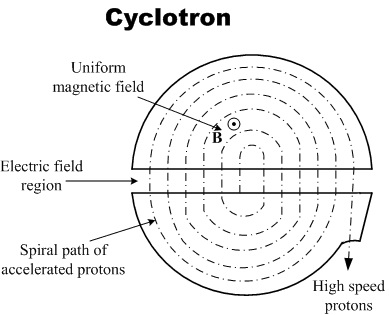# Proton inside a cyclotronConsider a standard cyclotron accelerator in which two semi-circular regions (dees) are connected to an AC voltage source which provides an electric field $E$ and a uniform vertical (out of the page) magnetic $B$ field inside cyclotron which is perpendicular to the electric field. The electric field switches such that is always points toward the dee the particle is not in.

A proton is released from rest such that it starts rotating in the cyclotron at radius $R$ and finally comes out from the slits of the cyclotron. The distance between the two semi-circular regions is $d$. Find the maximum number of turns proton take before coming out from slit's.

Details and Assumptions

${ { m }_{ p }=1.6\times { 10 }^{ -27 }\text{ kg}\\ { q }_{ p }=1.6\times { 10 }^{ -19 } C\\ B={ 10 }^{ -4 }\text{ T}\\ R=6\text{ m}\\ E=10\frac{\text{V}}{\text{m}}\\ d=10\text{ cm}\\ }$.

×